CBSE Class 10 Sample Paper for 2018 Boards

Class 10
Solutions of Sample Papers for Class 10 Boards

### The points A(4, –2), B(7, 2), C(0, 9) and D(–3, 5) form a parallelogram. Find the length of the altitude of the parallelogram on the base AB.

This is a question of CBSE Sample Paper - Class 10 - 2017/18.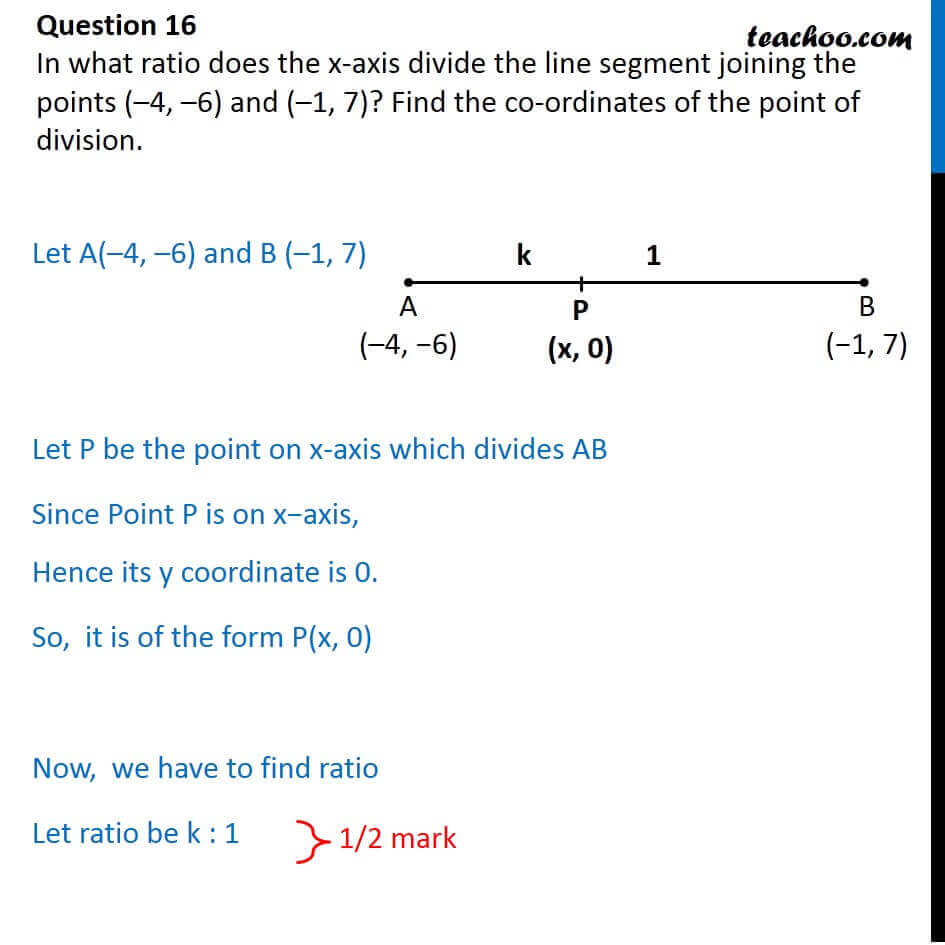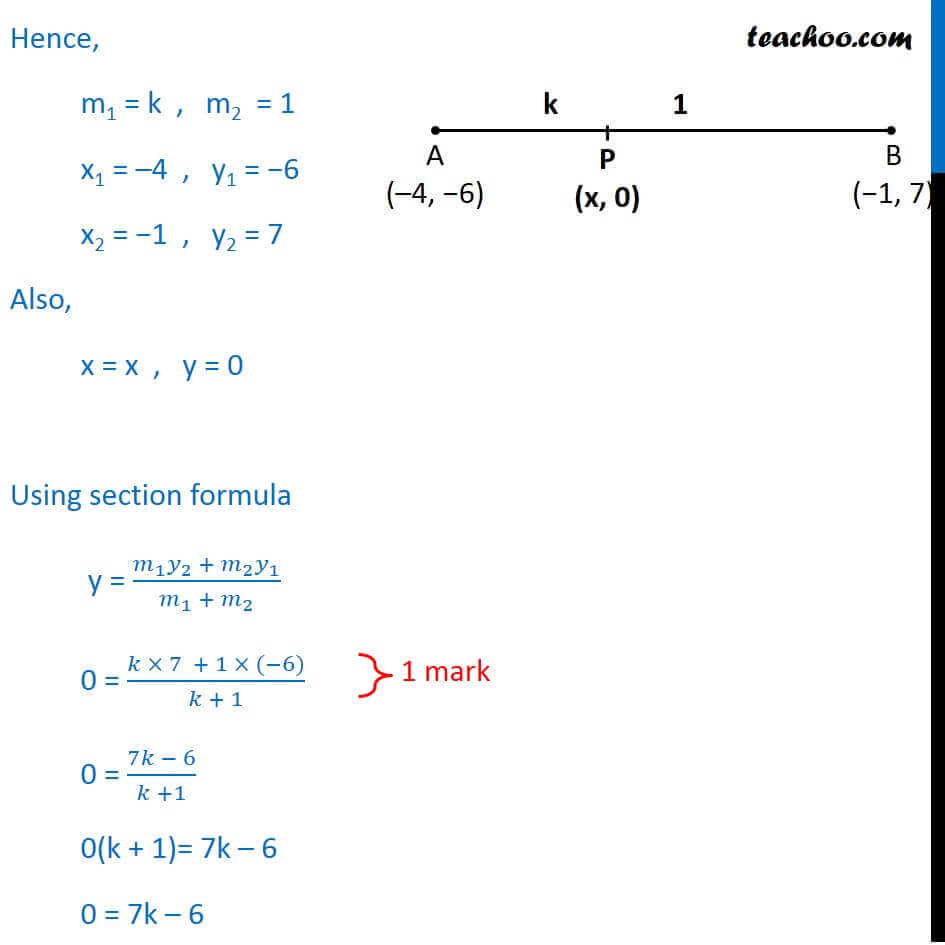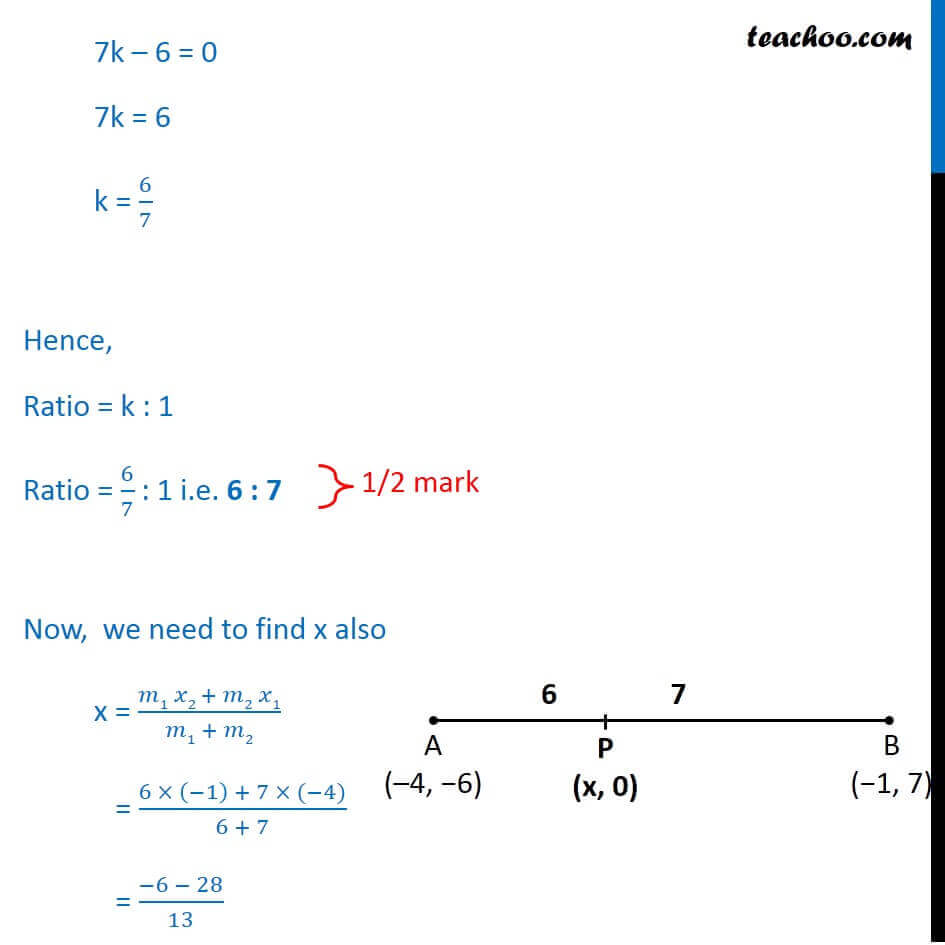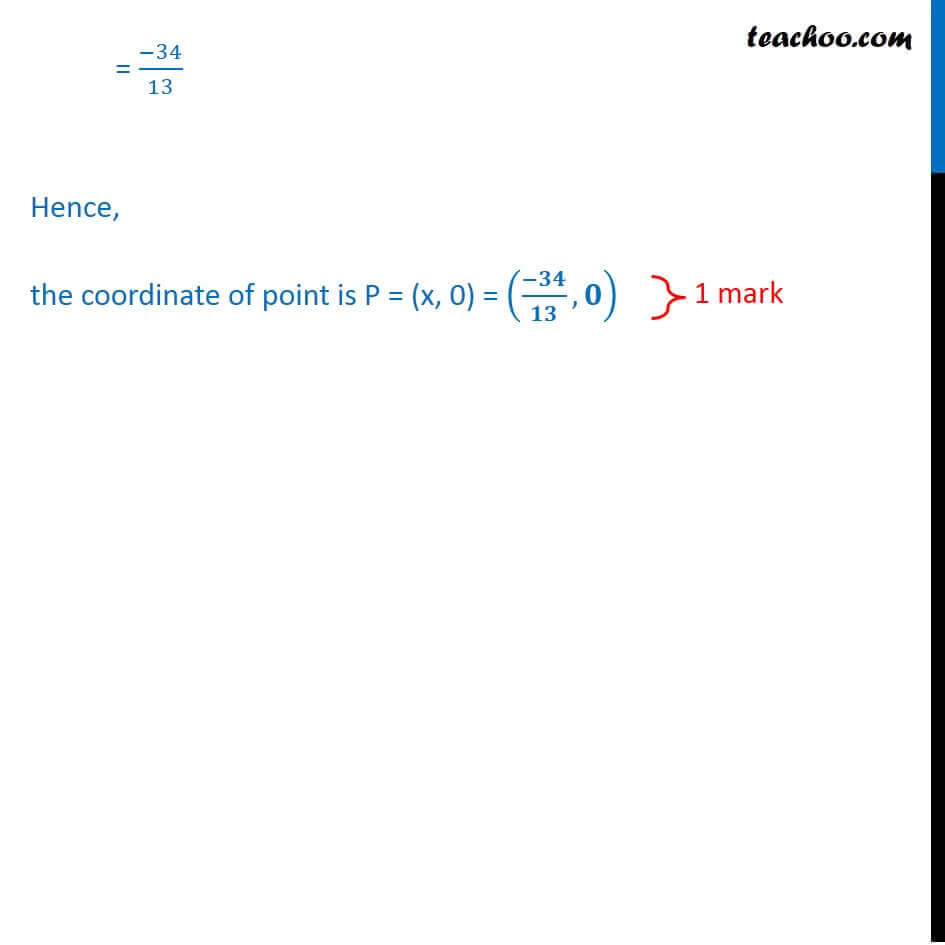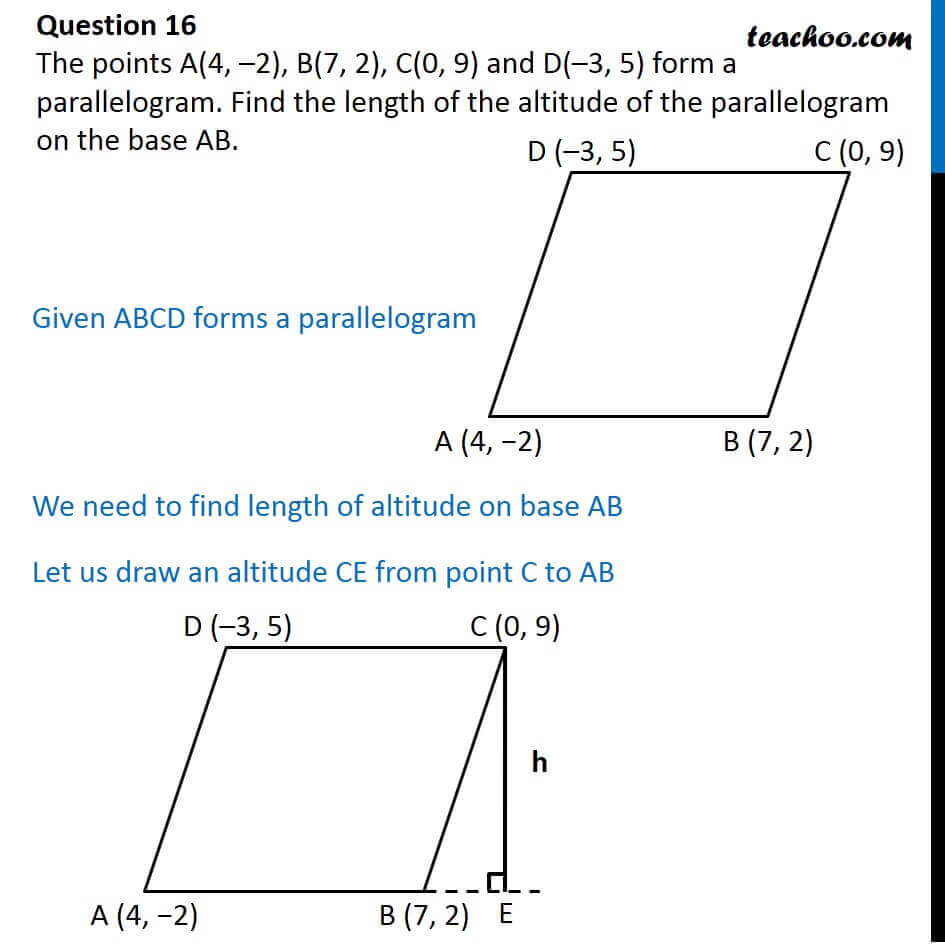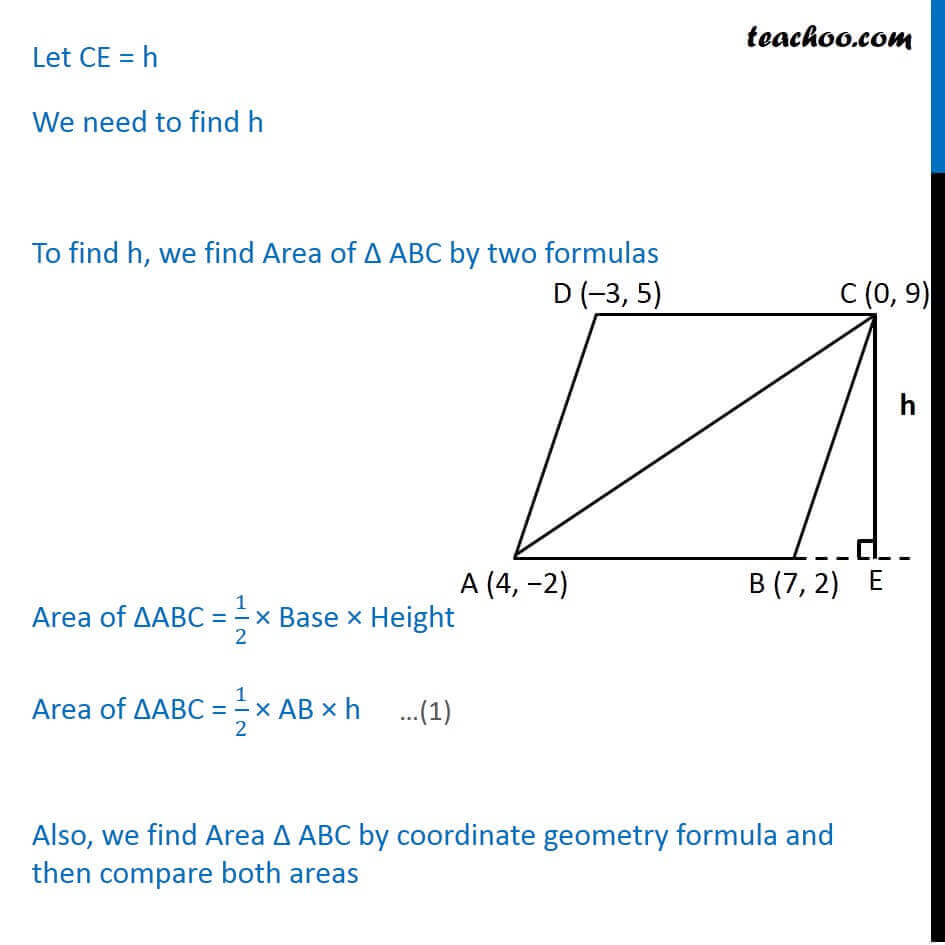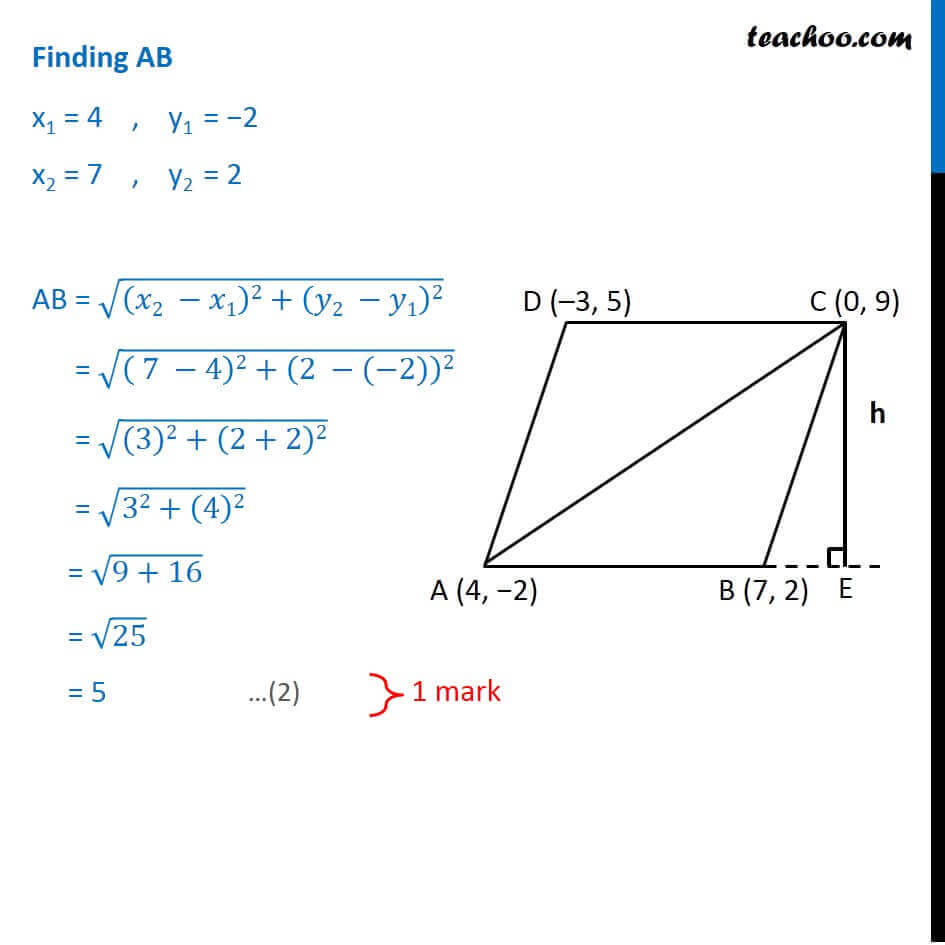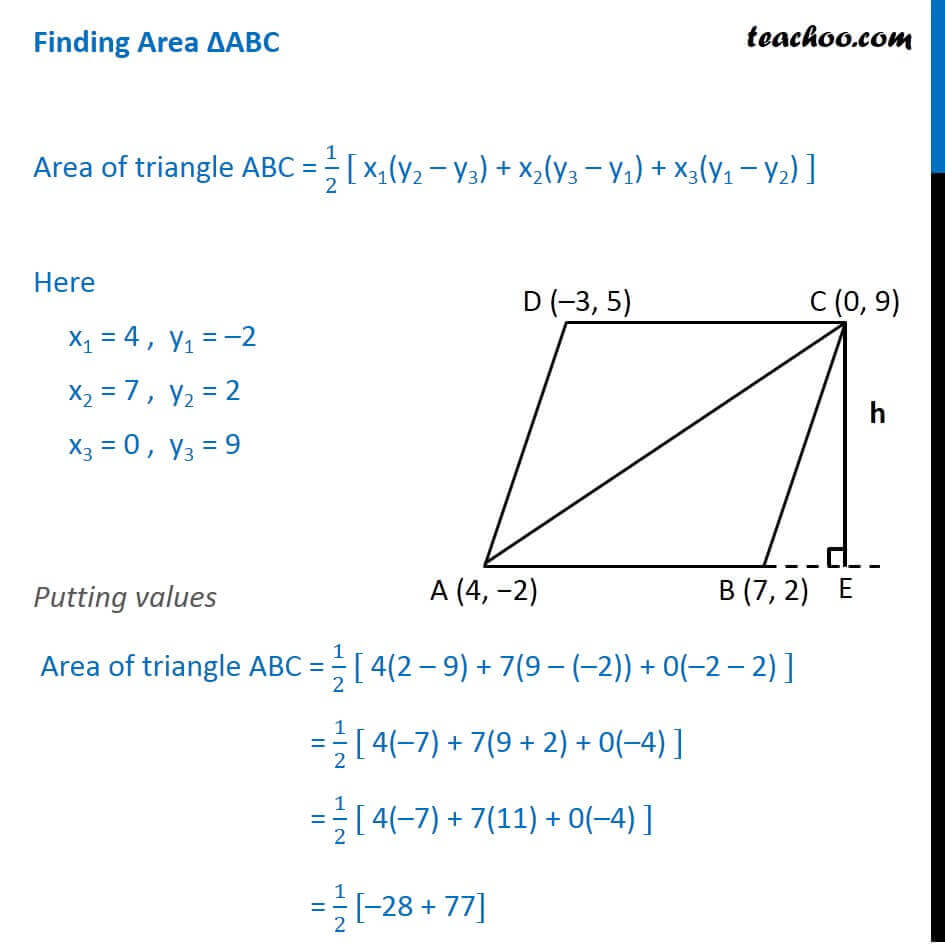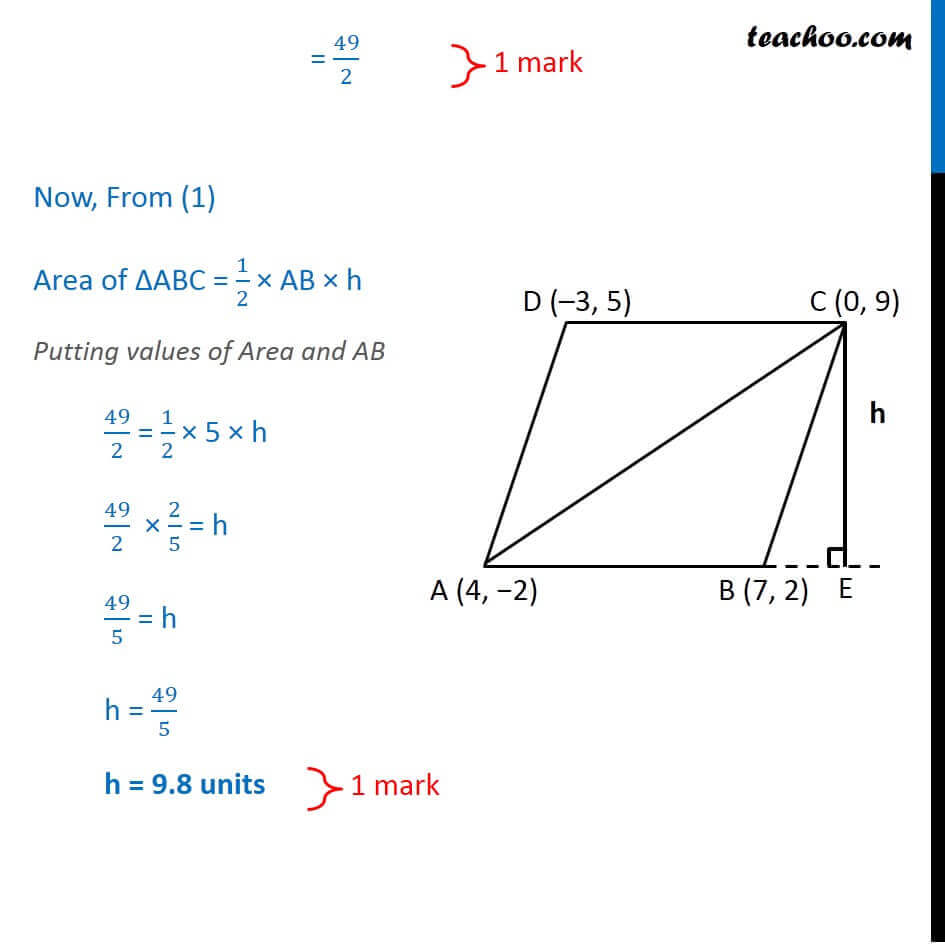Get live Maths 1-on-1 Classs - Class 6 to 12

### Transcript

Question 16 In what ratio does the x-axis divide the line segment joining the points ( 4, 6) and ( 1, 7)? Find the co-ordinates of the point of division. Let A( 4, 6) and B ( 1, 7) Let P be the point on x-axis which divides AB Since Point P is on x axis, Hence its y coordinate is 0. So, it is of the form P(x, 0) Now, we have to find ratio Let ratio be k : 1 Hence, m1 = k , m2 = 1 x1 = 4 , y1 = 6 x2 = 1 , y2 = 7 Also, x = x , y = 0 Using section formula y = ( _1 _2 + _2 _1)/( _1 + _2 ) 0 = ( 7 + 1 ( 6))/( + 1) 0 = (7 6)/( +1) 0(k + 1)= 7k 6 0 = 7k 6 7k 6 = 0 7k = 6 k = 6/7 Hence, Ratio = k : 1 Ratio = 6/7 : 1 i.e. 6 : 7 Now, we need to find x also x = ( 1 2 + 2 1)/( 1 + 2) = (6 ( 1) + 7 ( 4))/(6 + 7) = ( 6 28)/13 = ( 34)/13 Hence, the coordinate of point is P = (x, 0) = (( )/ , ) Question 16 The points A(4, 2), B(7, 2), C(0, 9) and D( 3, 5) form a parallelogram. Find the length of the altitude of the parallelogram on the base AB. Given ABCD forms a parallelogram We need to find length of altitude on base AB Let us draw an altitude CE from point C to AB Let CE = h We need to find h To find h, we find Area of ABC by two formulas Area of ABC = 1/2 Base Height Area of ABC = 1/2 AB h Also, we find Area ABC by and then compare both areas Finding AB x1 = 4 , y1 = 2 x2 = 7 , y2 = 2 AB = (( 2 1)2+( 2 1)2) = (( 7 4)2+(2 ( 2))2) = ((3)2+(2+2)2) = (32+(4)2) = (9+16) = 25 = 5 Finding Area ABC Area of triangle ABC = 1/2 [ x1(y2 y3) + x2(y3 y1) + x3(y1 y2) ] Here x1 = 4 , y1 = 2 x2 = 7 , y2 = 2 x3 = 0 , y3 = 9 Putting values Area of triangle ABC = 1/2 [ 4(2 9) + 7(9 ( 2)) + 0( 2 2) ] = 1/2 [ 4( 7) + 7(9 + 2) + 0( 4) ] = 1/2 [ 4( 7) + 7(11) + 0( 4) ] = 1/2 [ 28 + 77] = 49/2 Now, From (1) Area of ABC = 1/2 AB h Putting values of Area and AB 49/2 = 1/2 5 h 49/2 2/5 = h 49/5 = h h = 49/5 h = 9.8 units Question 16 The points A(4, 2), B(7, 2), C(0, 9) and D( 3, 5) form a parallelogram. Find the length of the altitude of the parallelogram on the base AB. Given ABCD forms a parallelogram We need to find length of altitude on base AB Let us draw an altitude CE from point C to AB Let CE = h We need to find h To find h, we find Area of ABC by two formulas Area of ABC = 1/2 Base Height Area of ABC = 1/2 AB h Also, we find Area ABC by coordinate geometry formula and then compare both areas Finding AB x1 = 4 , y1 = 2 x2 = 7 , y2 = 2 AB = (( 2 1)2+( 2 1)2) = (( 7 4)2+(2 ( 2))2) = ((3)2+(2+2)2) = (32+(4)2) = (9+16) = 25 = 5 Finding Area ABC Area of triangle ABC = 1/2 [ x1(y2 y3) + x2(y3 y1) + x3(y1 y2) ] Here x1 = 4 , y1 = 2 x2 = 7 , y2 = 2 x3 = 0 , y3 = 9 Putting values Area of triangle ABC = 1/2 [ 4(2 9) + 7(9 ( 2)) + 0( 2 2) ] = 1/2 [ 4( 7) + 7(9 + 2) + 0( 4) ] = 1/2 [ 4( 7) + 7(11) + 0( 4) ] = 1/2 [ 28 + 77] = 49/2 Now, From (1) Area of ABC = 1/2 AB h Putting values of Area and AB 49/2 = 1/2 5 h 49/2 2/5 = h 49/5 = h h = 49/5 h = 9.8 units